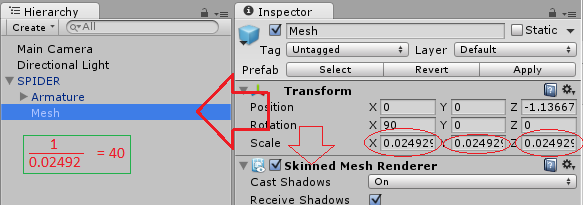# How to scale Mesh.bindPose correctly

Hello everyone,

I’m stuck with a mathematical problem. I want to set all bones of a model to their bind poses. This works OK for most of the models but there is one where the scale in the bind pose is 40 (I guess it was created using that scale, and after importing in Unity it got scaled down automatically to 1). So I want to scale the whole bindPose array down so that every bone transform has a scale of 1 (to match my default scale). But this doesn’t work as expected (the whole mesh get’s messed up).

``````Matrix4x4 bindPose = skinnedMeshRenderer.sharedMesh.bindposes[boneIndex];

// Scale by 1 / 40 so that the end result is 1
Vector3 scale = new Vector3(1/40, 1/40, 1/40);
Matrix4x4 normalizeScale = Matrix4x4.Scale(scale);

// Apply the resulting matrix afterwards
Matrix4x4 result = transform.localToWorldMatrix * normalizeScale * bindPose.inverse;
``````

So I guess either the order in which I multiply the matrices is not correct, or I’m doing the scale in the wrong space?

Your calculation doesn’t make much sense ^^. The bindpose matrix is a matrix that should transform a point from the mesh’s local space into the localspace of the bone. Once in that space Unity’s animation system would then transform the position from the bones local space into world space using the current localToWorld matrix of the bone.

Next thing is this won’t work:

``````Vector3 scale = new Vector3(1/40, 1/40, 1/40);
``````

this will give you a (0, 0, 0) vector because 1 and 40 are both integer values.

``````Matrix4x4 normalizeScale = Matrix4x4.Scale(Vector3.one / 40f);
``````

Since it’s the bones local space which is wrongly scaled you want the scaling to happen after all the other transformations. So you have to put your matrix infront of your original bindpose:

``````bindPose = normalizeScale * bindPose;
``````

Next thing is it’S not clear what you do with your “result”. Keep in mind that `sharedMesh.bindposes` is a property, so you can’t assign seperate matrices to that array. You have to use a temp array, modify your matrices and finally assign the whole array back to sharedMesh.bindposes.

Final Note: Messing with bindposes of imported models isn’t a good idea. It will most likely mess up all animations you have. If there is an issue in the model itself, it should be fixed in the modelling tool.

If you just want to change the scale factor of the model, check out the model inspector of the imported model. You can specify a scale factor there. It will reimport the model and will guarantee that everything will still work (as long as the model doesn’t have an error already ^^).

edit
Ahh, thanks to your comment i think we can solve that problem ^^. Your mistake is that you used the Amature transform. You have to use the mesh transform.The mesh is actually rendered by the SkinnedMeshRenderer. So the transform of the object with the SkinnedMeshRenderer is the one you have to use when you revert the bindpose.

So the original bindpose matrix converts the local vertex coordinates from the local mesh space into the local space of the bone. When you invert the matrix you convert from bone to local mesh space. So then using the localToWorld matrix of the SkinnedMeshRenderer you would get back the bone matrix.

This forum post by hippocoder seems to be relevant.